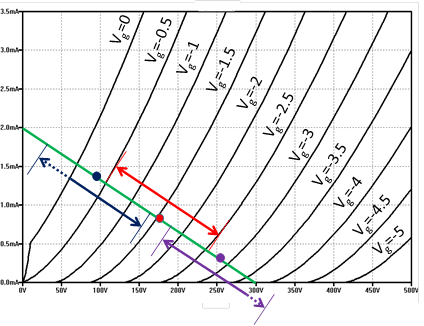The operating point of a vacuum tube is the position on the loadline, corresponding to the voltage and current measured at the anode in the quiescent status, that is when no signal is applied to the grid. The operating point is set by providing the grid with an appropriate fixed negative voltage Vg, with respect to the cathode. This fixed voltage is commonly referred as the grid bias voltage, or simply as the bias, of the vacuum tube.

Using the average anode characteristic graph and the loadline, the operating point is identified by the intersection of the loadline with the plot corresponding to the chosen grid bias voltage Vg. Let us use Figure 8 and the green loadline to exemplify this. Suppose that the grid bias voltage Vg is set to -1,5V. In this case, the operating point is the red spot, at the intersection between the green loadline and the plot, corresponding to a grid voltage Vg of -1.5V. This corresponds to a quiescent anode voltage Vq of 175V and a quiescent anode current of 0.85 mA.

Suppose now that an input signal Vin ranging between +1 and -1V is applied to the grid. The voltage seen at the grid is Vg+Vin and ranges between -0.5 and -2.5V. This produces a corresponding oscillation along the loadline, around the operating point, as depicted by the red range in the figure. The voltage measured at the anode is Vq+Vout and oscillates, roughly, in the range between 110V and 240V, that is Vout ranges from -65 to +65V.

If the operating point is set too high, that is if the grid bias voltage is too high, as exemplified by the blue spot and range, then saturation would occur when the signal oscillation produces a grid voltage above 0V. In fact, when grid voltage is zero, the vacuum tube has the maximum conduction, and no additional current is possible. On the other hand, if the bias is set too low, as depicted by the violet spot and range, when the voltage seen at the grid is too low, the vacuum tube would quit conducting.

Note that, as better discussed in Section 3.5, there are cases where it is not needed to amplify the entire input signal and the operating point is set on purpose so that just a portion of the signal is correctly amplified.Figure 8: Operating point of a vacuum tube voltage amplifier.If the operating point is too high (blue spot and blue range), large signals applied to the grid produce saturation, since when the grid voltage is 0 we have the maximum conduction between cathode and anode. On the other hand, when the operating point is too low (violet spot and violet range), too small signals bring to zero conduction between cathode and anode. The operating point also determines the linearity response of the vacuum tube. If the operating point is correctly chosen (for instance, red spot and red range), the vacuum tube responds linearly to the entire voltage range applied to the grid.

### 3.3.1    Linearity of the amplifier

The optimal choice of the loadline, and the quiescent operating point, should identify a range where the vacuum tube operates linearly. Linear operation means that variations of the voltage applied to the grid should be linearly reflected in variations along the loadline. Graphically, this means that the black plots, corresponding to different grid voltages, should be uniformly distributed along the portion of the loadline used. For instance, in Figure 8, the intersections between the black plots and the green loadline are denser in correspondence of value of the grid voltage around -3.0V, rather than voltages around -1.0V. This means that, when the grid voltage varies around -3.0V, it produces variation of the anode voltage smaller than when the grid voltage varies around -1.0V. If the grid is provided with a signal that spans from -1.0V to -3.0V, this is not linearly amplified and harmonic distortions are generated.